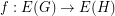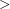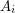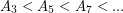# Antichains in the cycle continuous order

 Importance: Medium ✭✭
 Author(s): DeVos, Matt
 Subject: Graph Theory » Coloring » » Nowhere-zero flows
 Keywords: antichain cycle poset
 Recomm. for undergrads: no
 Prize: Bottle of wine (DeVos)
 Posted by: mdevos on: May 12th, 2007

If,are graphs, a functionis called cycle-continuous if the pre-image of every element of the (binary) cycle space ofis a member of the cycle space of.

Problem   Does there exist an infinite set of graphsso that there is no cycle continuous mapping betweenandwhenever?

The definition of a cycle-continuous mapping is based on some work of Jaeger, and the most interesting question on this subject is undoubtedly Jaeger's Petersen coloring conjecture.

Let us define a relation on the set of all finite graphs with at least one edge by the ruleif there is a cycle-continuous mapping fromto. It is not difficult to verify thatis a quasi order (reflexive and transitive). In this order, every Eulerian graph dominates every other graph, and every graph with a cut edge is dominated by every other graph.

Letbe the graph on two vertices withparallel edges. Thenwith all the inequalities strict, so this sequence is an infinite chain. Very little else seems to be known about this order. In particular, the problem highlighted above - does there exist an infinite antichain? remains open.

## Bibliography

* indicates original appearance(s) of problem.